Excel SUM Formula | CustomGuide# Excel SUM Formula

How to Use Sum and Average Formulas in Excel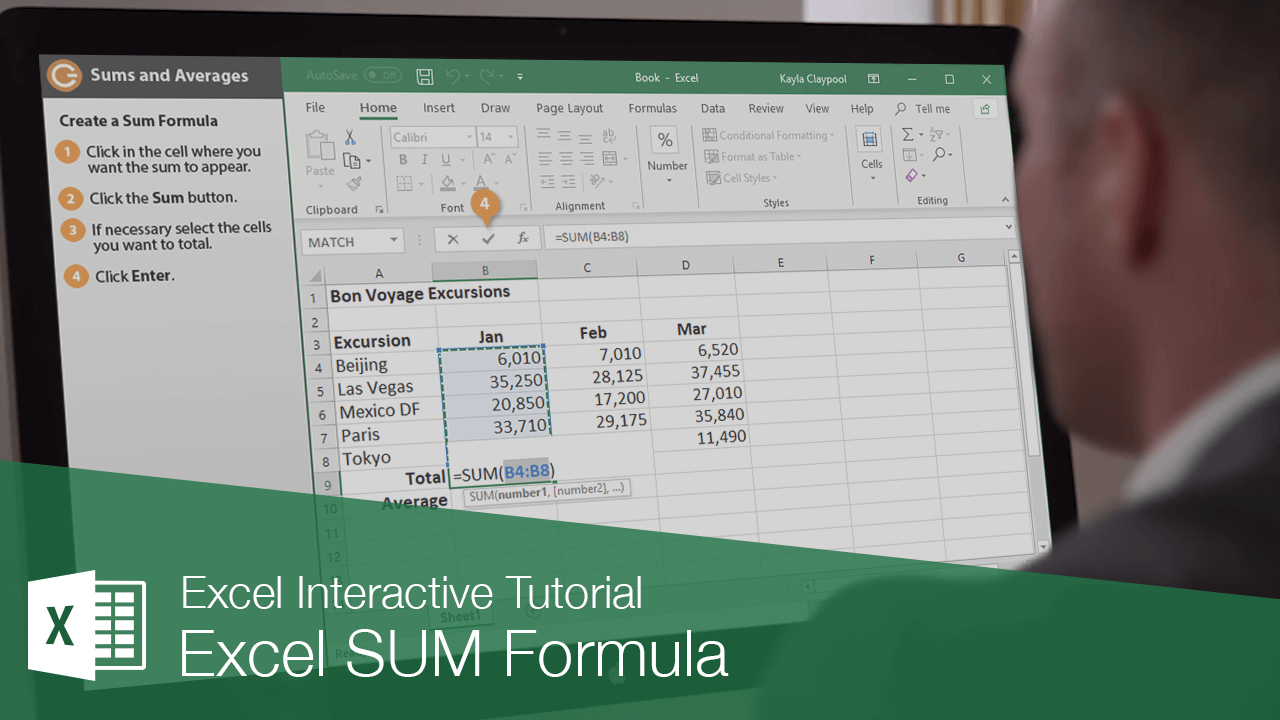One of the common Excel functions is SUM, which totals the values in a range of cells. For example, the formula =SUM(A2:A10) totals the values in the cell range A2:A10. The AVERAGE function is similar to SUM, except it finds the average of values in a cell range.

### Create a Sum Formula

Creating a sum formula is simple.

1. Click in the cell where you want the sum to appear.
2. Click the AutoSum button.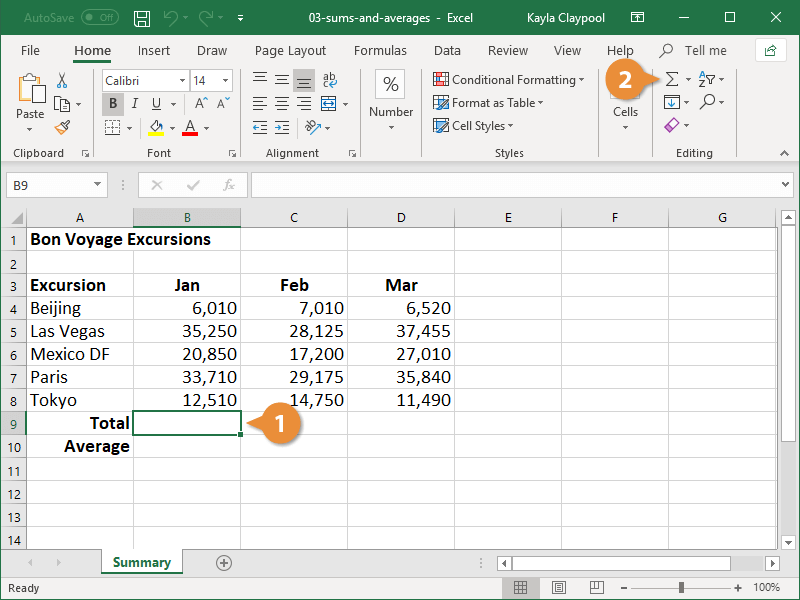3. If necessary, select the cells you want to total.

Excel will automatically select the cell range it thinks you want to total, but if this range is not correct you can select the correct cell range.

4. Click Enter or press the Enter key.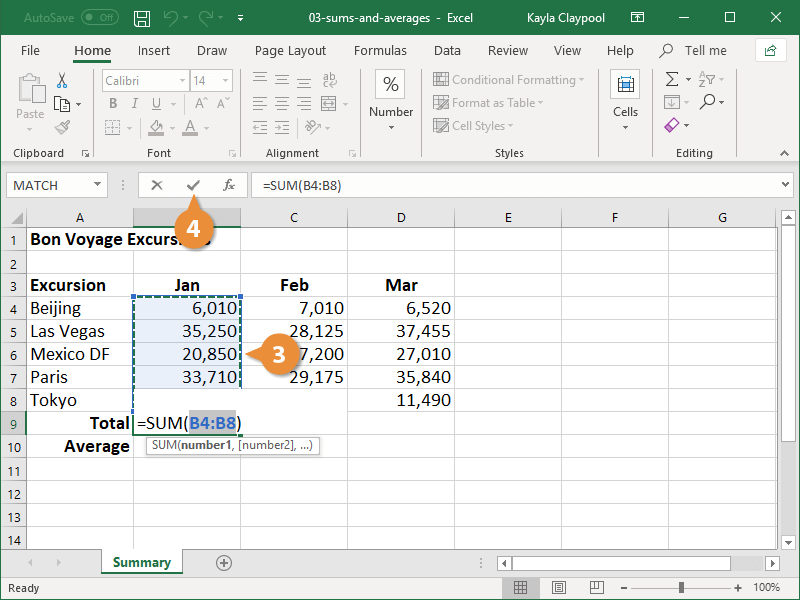You can also calculate a sum with your keyboard by pressing Alt + =.

The cell range is totaled in the cell. If you change a value in the summed range, the formula will automatically update to show the new sum.

### Create an Average Formula

The AVERAGE function works just like SUM, except it finds the average of a range of cells.

1. Click in the cell where you want the average to appear.
2. Click the AutoSum button list arrow.
3. Select Average.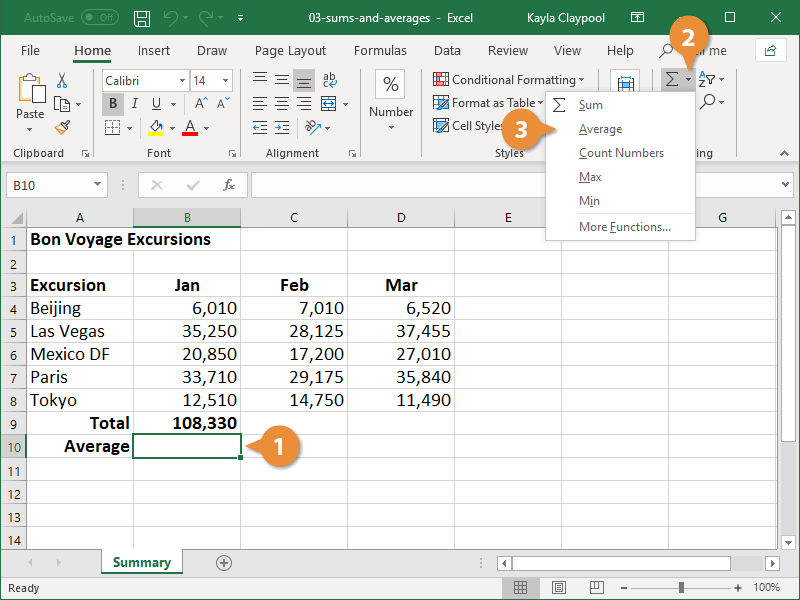4. If necessary, select the cells you want to total.
5. Click Enter or press the Enter key.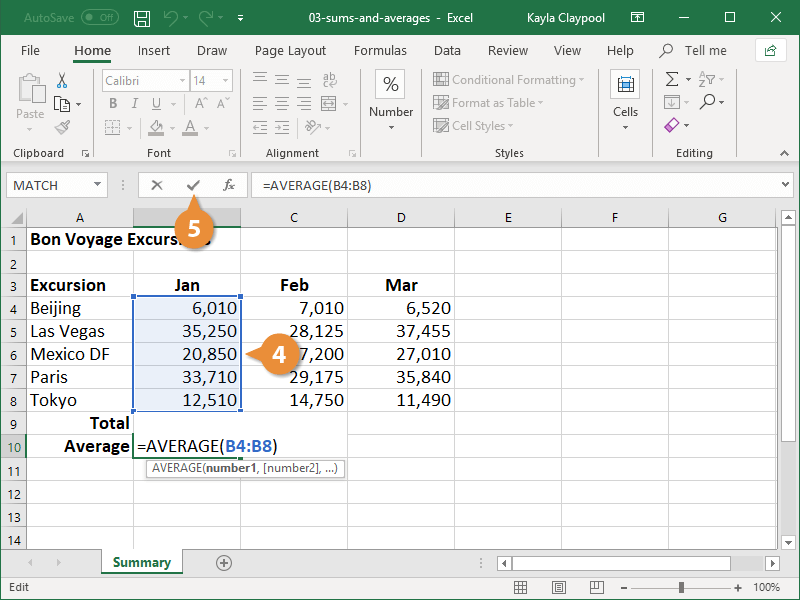The average of the cell range is displayed in the cell.